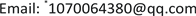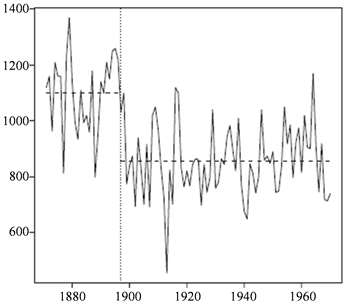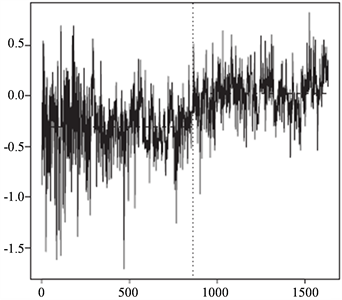﻿ 长记忆时间序列均值多变点滑动比检验及应用 Moving Ratio Test for Multiple Mean Changes in Long Memory Time Series with Applications

Statistics and Application
Vol.07 No.02(2018), Article ID:24322,11 pages
10.12677/SA.2018.72011

Moving Ratio Test for Multiple Mean Changes in Long Memory Time Series with Applications

Guolong Fu*, Mingcan He

Department of Mathematics and Statistics, Qinghai Normal University, Xining QinghaiReceived: Mar. 12th, 2018; accepted: Mar. 28th, 2018; published: Apr. 4th, 2018ABSTRACT

In this paper, by introducing a bandwidth parameter, a moving ratio type statistic is proposed to detect multiple mean changes in long memory time series. The null distribution of the test statistic and its consistency under the alternative hypothesis are proved. Simulations show that the proposed new method can effectively solve the problem of testing failure when using the single change point test method to test multiple change points. Finally, the practicability of the method is illustrated by two groups of actual data.

Keywords:Long Memory Time Series, Mean Change Point, Moving Ratio Statistics1. 引言

Hidalgo和Robinson  在假定变点位置已知的情况下，提出了一种检验长记忆时间序列均值变点的Wald方法，Wright  在变点位置未知的情况下，研究了长记忆时间序列中的均值变点，并提出了一种CUSUM检验方法和一种Sup-Wald检验方法。Lazarova  研究了线性回归模型含有长记忆时间序列误差项时结构变点的检验问题，Wang  研究了无限滑动平均时间序列均值变点的检验和估计问题，Shao  通过提出一种简单的比率型统计量继续研究长记忆时间序列均值变点的检验问题，这种比率型检验方法的有点是不需要估计尺度参数等冗余参数，在实际应用中非常方便。赵文芝等  ，马健琦等  提出了一些新的比率型统计量来检验长记忆时间序列中的均值变点，模拟结果表明这些新比率统计量具有比原比率型统计量更高的检验势。

2. 长记忆模型和检验统计量

${\left(1-B\right)}^{d}\left({Y}_{t}-\mu \right)={u}_{t}$ , $t=1,\cdots ,n$

${Y}_{t}=\sum _{j=0}^{\infty }{\pi }_{j}\left(d\right){u}_{t-j}$ , ${\pi }_{j}\left(d\right)=\frac{\Gamma \left(d+j\right)}{j!\Gamma \left(d\right)}$ , $j=1,2,\cdots$ , ${\pi }_{0}\left(d\right)=1$ .

${X}_{t}={\mu }_{t}+{Y}_{t}$ 其中 ${Y}_{t}\sim I\left(d\right)$$d\in \left[0,1/2\right)$ ，本文考虑平稳长记忆时间序列均值多变点的检验问题，即考虑如下假设检验问题：

${H}_{0}:{\mu }_{1}={\mu }_{2}=\cdots ={\mu }_{n}$

$\begin{array}{l}{H}_{1}:{\mu }_{1}=\cdots ={\mu }_{{k}_{1}-1}\ne {\mu }_{{k}_{1}}=\cdots ={\mu }_{{k}_{1}+{k}_{2}-1}\ne {\mu }_{{k}_{1}+{k}_{2}}=\cdots \\ \text{\hspace{0.17em}}\text{\hspace{0.17em}}\text{\hspace{0.17em}}\text{\hspace{0.17em}}\text{\hspace{0.17em}}\text{\hspace{0.17em}}\text{\hspace{0.17em}}\text{\hspace{0.17em}}\text{\hspace{0.17em}}\text{\hspace{0.17em}}={\mu }_{{k}_{1}+{k}_{2}+\cdots +{k}_{n-1}+1}={\mu }_{{k}_{1}+{k}_{2}+\cdots +{k}_{n}-1}\ne {\mu }_{{k}_{1}+{k}_{2}+\cdots +{k}_{n}}\end{array}$

${\mu }_{t}=\left\{\begin{array}{ll}{\mu }_{{k}_{1}},\hfill & t=1,2,\cdot \cdot \cdot ,{k}_{1}^{*}\hfill \\ {\mu }_{{k}_{1}+{k}_{2}},\hfill & t={k}_{1}^{*}+1,\cdot \cdot \cdot ,{k}_{2}^{*}\hfill \\ \text{\hspace{0.17em}}\text{\hspace{0.17em}}\text{\hspace{0.17em}}⋮\hfill & \text{\hspace{0.17em}}\text{\hspace{0.17em}}\text{\hspace{0.17em}}\text{\hspace{0.17em}}\text{\hspace{0.17em}}\text{\hspace{0.17em}}\text{\hspace{0.17em}}\text{\hspace{0.17em}}\text{\hspace{0.17em}}⋮\hfill \\ {\mu }_{{k}_{1}+{k}_{2}+\cdot \cdot \cdot +{k}_{n}},\hfill & t={k}_{m}^{*}+1,\cdot \cdot \cdot ,n\hfill \end{array}$

${\mu }_{t}=\left\{\begin{array}{ll}\mu ,\hfill & t=1,2,\cdot \cdot \cdot ,{k}_{1}^{*}\hfill \\ \mu +{\Delta }_{1},\hfill & t={k}_{1}^{*}+1,\cdot \cdot \cdot ,{k}_{2}^{*}\hfill \\ \text{\hspace{0.17em}}\text{\hspace{0.17em}}\text{\hspace{0.17em}}⋮\hfill & \text{\hspace{0.17em}}\text{\hspace{0.17em}}\text{\hspace{0.17em}}\text{\hspace{0.17em}}\text{\hspace{0.17em}}\text{\hspace{0.17em}}\text{\hspace{0.17em}}\text{\hspace{0.17em}}\text{\hspace{0.17em}}⋮\hfill \\ \mu +{\Delta }_{m},\hfill & t={k}_{m}^{*}+1,\cdot \cdot \cdot ,n\hfill \end{array}$ .

${H}_{0}$ : ${\Delta }_{1}={\Delta }_{2}=\cdots ={\Delta }_{m}=0$ ;

${H}_{1}$${\Delta }_{1},{\Delta }_{2},\cdots ,{\Delta }_{m}$ 至少有一个不为零。

${G}_{n}=\frac{\sqrt{n}|{\overline{X}}_{1,k}-{\overline{X}}_{k+1,n}|}{{\left(n\right)}^{-1}{\left\{{\sum }_{t-1}^{k}{S}_{t}^{2}\left(1,k\right)+{\sum }_{t=k+1}^{n}{S}_{t}^{2}\left(k+1,n\right)\right\}}^{1/2}}$ , $\text{1}\le k\le n$ (1)

${S}_{t}\left(j,k\right)={\sum }_{i=j}^{t}\left({X}_{i}-{\overline{X}}_{j,k}\right)$ , ${\overline{X}}_{j,k}=\frac{1}{k-j+1}{\sum }_{t=j}^{k}{X}_{t}$ , $t=j,\cdots ,k$ , $1\le j .

${H}_{L}\left(k\right)=\frac{\sqrt{2L}|{\overline{X}}_{k-L+1,k}-{\overline{X}}_{k+1,k+L}|}{{\left(2L\right)}^{-1}\left\{{\sum }_{t=k-L+1}^{k}{S}_{t}^{2}\left(k-L+1,k\right)+{{\sum }_{t=k+1}^{k+L}{S}_{t}^{2}\left(k+1,k+L\right)\right\}}^{1/2}}$ (2)

${n}^{-\left(1/2+d\right)}\sum _{t=1}^{⌊nr⌋}\left\{{X}_{t}-E\left({X}_{t}\right)\right\}⇒{C}_{d}{W}_{d}\left(r\right)$ , $r\in \left[0,1\right]$

3. 检验统计量的渐近性质

${H}_{L}\left(k\right)=\frac{\sqrt{2}|2{W}_{d}\left(r\right)-{W}_{d}\left(r-1/2\right)-{W}_{d}\left(r+1/2\right)|}{{\int }_{r-1/2}^{r}{\xi }_{1}^{2}\left(s,r\right){d}_{s}+{\int }_{r}^{r+1/2}{\xi }_{2}^{2}\left(s,r\right){d}_{s}}$

${\xi }_{1}\left(s,r\right)={W}_{d}\left(s\right)-\left(2s-2r+1\right){W}_{d}\left(r\right)+2\left(s-r\right){W}_{d}\left(r-1/2\right)$

${\xi }_{2}\left(s,r\right)={W}_{d}\left(s\right)-2\left(s-r\right){W}_{d}\left(r+1/2\right)+\left(2s-2r-1\right){W}_{d}\left(r\right)$

${2}^{-1/2-d}{L}^{1/2-d}\left({\overline{X}}_{k-L+1,k}-{X}_{k+1,k+L}\right)={\left(2L\right)}^{-1/2-d}\left[\sum _{t=k-L+1}^{k}\left({X}_{t}-E{X}_{t}\right)-\sum _{t=k+1}^{k+L}\left({X}_{t}-E{X}_{t}\right)\right]$

$⇒{C}_{d}\left[2{W}_{d}\left(r\right)-{W}_{d}\left(r-1/2\right)-{W}_{d}\left(r+1/2\right)\right]$

$\begin{array}{l}{\left(2L\right)}^{-1/2-d}{S}_{t}\left(k-L+1,k\right)={\left(2L\right)}^{-1/2-d}\sum _{i=k-L+1}^{t}\left({X}_{i}-\frac{1}{L}\sum _{j=k-L+1}^{k}{X}_{j}\right)\\ \text{ }={\left(2L\right)}^{-1/2-d}\left[\sum _{i=k-L+1}^{t}\left({X}_{i}-E{X}_{t}\right)-\frac{t-k+L}{L}\sum _{j=k-L+1}^{k}\left({X}_{j}-E{X}_{t}\right)\right]\end{array}$

$⇒{C}_{d}\left[{W}_{d}\left(s\right)-\left(2s-2r+1\right){W}_{d}\left(r\right)+2\left(s-r\right){W}_{d}\left(r-1/2\right)\right]\triangleq {C}_{d}{\xi }_{1}\left(s,r\right)$

${\left(2L\right)}^{-1/2-d}{S}_{t}\left(k+1,k+L\right)={\left(2L\right)}^{-1/2}\left[\sum _{i=k+1}^{t}\left({X}_{i}-E{X}_{t}\right)-\frac{t-k}{L}\sum _{j=k+1}^{k+L}\left({X}_{j}-E{X}_{t}\right)\right]$

$⇒{C}_{d}\left[{W}_{d}\left(s\right)-2\left(s-r\right){W}_{d}\left(r+1/2\right)+\left(2s-2r-1\right){W}_{d}\left(r\right)\right]\triangleq {C}_{d}{\xi }_{2}\left(s,r\right)$

$\begin{array}{l}{H}_{L}\left(k\right)=\frac{\sqrt{2L}|{\overline{X}}_{k-L+1,k}-{\overline{X}}_{k+1,k+L}|}{{\left(2L\right)}^{-1}\left\{{\sum }_{t=k-L+1}^{k}{S}_{t}^{2}\left(k-L+1,k\right)+{{\sum }_{t=k+1}^{k+L}{S}_{t}^{2}\left(k+1,k+L\right)\right\}}^{1/2}}\\ =\frac{{2}^{1+d}{L}^{d}|{2}^{-1/2-d}{L}^{1/2-d}\left({\overline{X}}_{k-L+1,k}-{\overline{X}}_{k+1,k+L}\right)|}{{2}^{1/2+d}{L}^{d}{\left\{{\left(2L\right)}^{-1}{\sum }_{t=k-L+1}^{k}{\left[{\left(2L\right)}^{-1/2-d}{S}_{t}\left(k-L+1,k\right)\right]}^{2}+{\left(2L\right)}^{-1}{\sum }_{t=k+1}^{k+L}{\left[{\left(2L\right)}^{-1/2-d}{S}_{t}\left(k+1,k+L\right)\right]}^{2}\right\}}^{1/2}}\end{array}$

$⇒\frac{\sqrt{2}|2{W}_{d}\left(r\right)-{W}_{d}\left(r-1/2\right)-{W}_{d}\left(r+1/2\right)|}{{\int }_{r-1/2}^{r}{\xi }_{1}^{2}\left(s,r\right){d}_{s}+{\int }_{r}^{r+1/2}{\xi }_{2}^{2}\left(s,r\right){d}_{s}}$ 证毕。

$\underset{L\to \infty }{\mathrm{lim}}{H}_{L}\left(k\right){\to }_{p}\infty$ .

${H}_{L}\left(k\right)=\frac{\sqrt{2L}|{\overline{X}}_{{k}^{*}-L+1,{k}^{*}}-{\overline{X}}_{{k}^{*}+1,{k}^{*}+L}|}{{\left(2L\right)}^{-1}\left\{{\sum }_{t={k}^{*}-L+1}^{{k}^{*}}{S}_{t}^{2}\left({k}^{*}-L+1,{k}^{*}\right)+{{\sum }_{t=k+1}^{k+L}{S}_{t}^{2}\left({k}^{*}+1,{k}^{*}+L\right)\right\}}^{1/2}}$

${S}_{t}\left({k}^{\ast }-L+1,{k}^{\ast }\right)={O}_{p}\left({\left(2L\right)}^{1/2+d}\right)$

${S}_{t}\left({k}^{\ast }+1,{k}^{\ast }+L\right)={O}_{p}\left({\left(2L\right)}^{1/2+d}\right)$

$\begin{array}{l}\sqrt{2L}|{\overline{X}}_{{k}^{\ast }-L+1,{k}^{\ast }}-{\overline{X}}_{{k}^{\ast }+1,{k}^{\ast }+L}|\\ \ge \sqrt{2L}|\frac{1}{L}\sum _{t={k}^{\ast }-L+1}^{{k}^{\ast }}\left({X}_{t}-\mu \right)-\frac{1}{L}\sum _{t={k}^{\ast }+1}^{{k}^{\ast }+L}\left({X}_{t}-\mu -\Delta \right)|+\sqrt{2L}|L\Delta |\\ ={I}_{1}+{I}_{2}\end{array}$

$\begin{array}{c}{2}^{-\left(1+d\right)}{L}^{-d}{I}_{1}={2}^{-\left(1+d\right)}{L}^{-d}\sqrt{2L}|\frac{1}{L}\sum _{t={k}^{\ast }-L+1}^{{k}^{\ast }}\left({X}_{t}-\mu \right)-\frac{1}{L}\sum _{t={k}^{\ast }+1}^{{k}^{\ast }+L}\left({X}_{t}-\mu -\Delta \right)|\\ =|{\left(2L\right)}^{-1/2-d}\sum _{t={k}^{\ast }-L+1}^{{k}^{\ast }}\left({X}_{t}-\mu \right)-{\left(2L\right)}^{-1/2-d}\sum _{t={k}^{\ast }+1}^{{k}^{\ast }+L}\left({X}_{t}-\mu -\Delta \right)|\end{array}$

$⇒{C}_{d}\left[2{W}_{d}\left({r}^{\ast }\right)-{W}_{d}\left({r}^{\ast }-1/2\right)-{W}_{d}\left({r}^{\ast }+1/2\right)\right]$

${I}_{1}={O}_{p}\left({2}^{1+d}{L}^{d}\right)$ 。而 ${I}_{2}=\sqrt{2L}|L\Delta |={O}_{p}\left({L}^{2}\right)$ 。因此，当 $n\to \infty$ ，即 $L\to \infty$ 时，有 ${H}_{L}\left(k\right)\to \infty$ 。证毕。

4. 数值模拟Table 1. Empirical size of the two statistics at the 5% test levelTable 2. Number of modified Shao statistics at change points m = 1 and empirical power at the 5% levelTable 3. Number of Shao statistics at change points m=1 and empirical power at the 5% level

5. 实例分析Table 4. Number of Shao statistics at change points m = 2 and empirical power at the 5% level

6. 总结Table 5. Number of Shao statistics at change points m = 2 and empirical power at the 5% levelFigure 1. Annual flow time series of the Nile Aswan DamFigure 2. Time series of annual temperature data in the northern hemisphere

Moving Ratio Test for Multiple Mean Changes in Long Memory Time Series with Applications[J]. 统计学与应用, 2018, 07(02): 79-89. https://doi.org/10.12677/SA.2018.72011

1. 1. Hidalgo, J. and Robinson, P.M. (1996) Testing for Structural Change in a Long-Memory Environment. Journal of Economerics, 70, 159-174. https://doi.org/10.1016/0304-4076(94)01687-9

2. 2. Wright, J.H. (2010) Testing for a Structural Break at Un-known Date with Long-Memory Disturbances. Journal of Time Series Analysis, 19, 369-376. https://doi.org/10.1111/1467-9892.00097

3. 3. Štěpána, L. (2005) Testing for Structural Change in Regression with Long Memory Processes. Journal of Econometrics, 129, 329-372. https://doi.org/10.1016/j.jeconom.2004.09.011

4. 4. Wang, L. (2008) Change-in-Mean Problem for Long Memory Time Series Models with Applications. Journal of Statistical Computation & Simulation, 78, 653-668. https://doi.org/10.1080/00949650701216604

5. 5. Shao, X. (2011) A Simple Test of Changes in Mean in the Possible Presence of Long-Range Dependence. Journal of Time Series Analysis, 32, 598-606. https://doi.org/10.1111/j.1467-9892.2010.00717.x

6. 6. 赵文芝, 夏志明, 贺飞跃. 长相依序列均值变点检测[J]. 数学的实践与认识, 2016, 46(3): 204-208.

7. 7. 马健琦, 陈占寿, 吕娜. 基于Sieve Bootstrap方法的长记忆过程均值变点的检验[J]. 青海师范大学学报(自科版), 2017, 33(2): 34-38.

8. 8. Kejriwal, M. and Zhou, J. (2013) Wald Tests for Detecting Multiple Struc-tural Changes in Persistence. Econometric Theory, 29, 289-323. https://doi.org/10.1017/S0266466612000357

9. 9. Bai, J. (1999) Likelihood Ratio Tests for Multiple Structural Changes. Journal of Econometrics, 91, 299-323. https://doi.org/10.1016/S0304-4076(98)00079-7

10. 10. 秦瑞兵, 田铮, 陈占寿. 独立随机序列均值多变点的非参数检测[J]. 应用概率统计, 2013, 29(5): 449-457.

11. 11. Chen, Z., Tian, Z., et al. (2012) Moving Ratio Test for Multiple Changes in Persis-tence. Journal of Systems Science & Complexity, 25, 582-593. https://doi.org/10.1007/s11424-012-9255-9

12. 12. Chen, Z., Jin, Z., Tian, Z., et al. (2012) Bootstrap Testing Multiple Changes in Persistence for a Heavy-Tailed Sequence. Computational Statistics & Data Analysis, 56, 2303-2316. https://doi.org/10.1016/j.csda.2012.01.011

13. NOTES

*通讯作者。